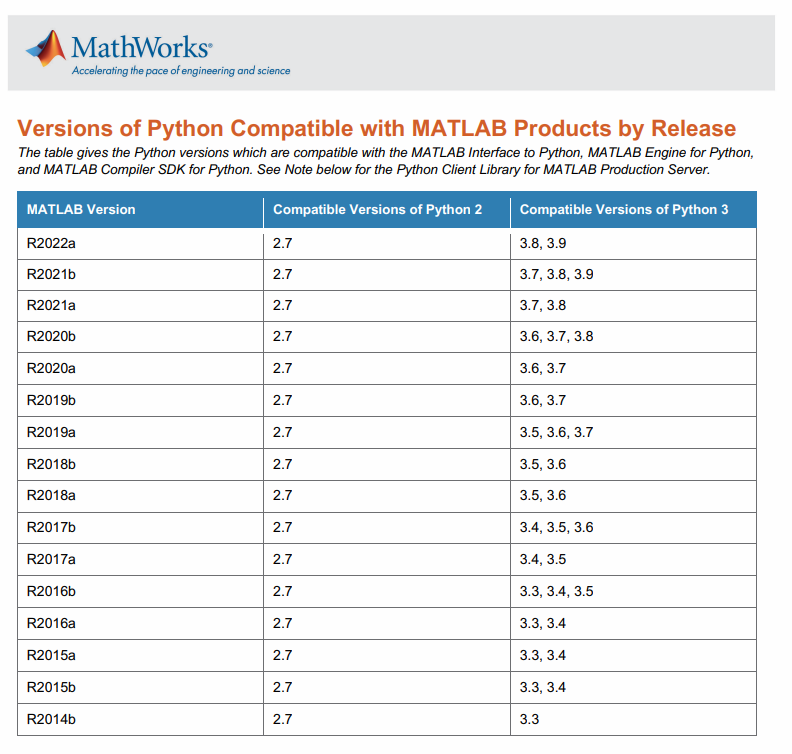# 一。主要流程

## 1.检查环境

``````% 检查Matlab能否调用Python
> pyversion

%如果不能调用python，可以给定python的可执行路径。如：
> pyversion('f:\Anaconda3\python.exe')
% 上面路径根据情况更改即可。
``````## 2. 调用python函数def或类class

• 建议正式调用前，先在matlab中添加路径（也就是.py文件所在位置）：
``````% 添加python的搜索路径
P = py.sys.path;
if count(P,'E:/XXX/XXX.py') == 0
insert(P,int32(0),'E:/XXX/XXX.py');
end
``````
• 然后在matlab中测试.py文件（也就是模块module）是否包含错误：
``````% 假设调用的.py文件的名称为：myfun.py
>> py.importlib.import_module('myfun')
``````

··· · `from .bootstrap import BootstrapResult`

···· 有一些图片输出或者bar显示的 函数，可能需要注释掉。否则也容易出错。

• 调用函数
假如我的.py文件为：
``````# myfun.py
c = a + b
return c
``````

``````% 在matlab中输入下面语句，调用.py的add函数
``````
• 调用类
假如我的.py文件为：
``````class myclass():
def __init__(self):
self.count = 0
def fit(self,X):
XXX 代码省略  XXX
print('count = ', self.count)
return G
``````

``````% 前面的测试
py.importlib.import_module('myfun')

% 需要实例化类。其中，myfun为.py文件的名称，myclass类名。
myRCD = py.myfun.myclass;

% 根据需要将输入的数据 转换为一维的numpy形式，方便调用。
X = py.numpy.array(X(:).');

% 调用实例化类myRCD的函数 fit
myRCD.fit(X);

% 根据需要把输出换成matlab的形式
``````

## 3. 补充

``````clear classes;
obj = py.importlib.import_module('rcd');
``````

## 现在的问题

• 无法解析名称 py.myfunc
-> 参考官网的链接 一般都能解决问题。
【https://ww2.mathworks.cn/help/matlab/matlab_external/undefined-variable-py-or-function-py-command.html】

# 以前遇到的问题

• UTF-8不能够编码的问题。
可能是多次在不同地方打开.py文件，导致其编码格式乱了。所以在当前环境下，能运行但是不能调试了。 后来，在anaconda里重新建立了一个新的Python环境，并在pycharm中打开.py文件，就可以直接运行调试了。所以，试试换环境看看？

具体操作大致是：打开cmd，来到当前.py文件所在的位置，使用anaconda下创建环境的方法创建，并激活。然后再用pycharm打开.py文件，设置project interpreter为当前建的环境。即可。
这里说的不是很详细，可以参考网上详细的步骤。

• 未定义.py或者类 “*****”
问题具体为：Importing the multiarray numpy extension module failed. Most likely you are trying to import a failed build of numpy.
If you’re working with a numpy git repo, try `git clean -xdf` (removes all files not under version control). Otherwise reinstall numpy.
Original error was: DLL load failed:
但是我们有一个方法可以解决：
请记得，要打开anaconda下的anaconda prompt，进入.py文件所在的文件夹，输入matlab，enter打开matlab。这种方式是比较方便的，它能让matlab搜索到Python路径。注意：一定要进入.py文件所在的文件夹，否则matlab还是无法知道python的搜索路径。
如果不行，就将模块里涉及到的包都重新卸载 再安装一次。因为有可能是里面的包有损坏之类的原因。

• RuntimeWarning: overflow encountered in cosh
意思是cosh(x)中代入的x值太大了。

• 为什么python代码在spyder内能正常运行，得到合适的结果（非零矩阵）；但是matlab调用python时，输出的结果却都是零矩阵呢？
奇了怪了…

• 如果是包内嵌的函数出现了错误，一定要检查清楚自己调用模块时，输入以及输出参数是否设置准确。

• 利用Anaconda升级Spyder=4.0.0时，出现错误：condaerror cannot link a source that does not exist。

• SyntaxError: invalid syntax 说明一定是哪里有语法错误。请在该错误的行上，前前后后的代码都要检查一遍。可以就是少了或者多了一个括号 或冒号: 之类的。

• python的输出是tuple类型，如何转化为double型？

``````// Output is data(m*n, tuple)
data = cell(data);
X = double(py.array.array('d',py.numpy.nditer(data{1})));
X = reshape(X,[n,m]);
X = transpose(X);
``````

# References

 https://zhuanlan.zhihu.com/p/92081119
 https://ww2.mathworks.cn/help/matlab/matlab_external/undefined-variable-py-or-function-py-command.html
 https://www.mathworks.com/content/dam/mathworks/mathworks-dot-com/support/sysreq/files/python-compatibility.pdf